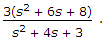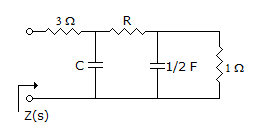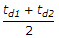# Electronics and Communication Engineering - Networks Analysis and Synthesis

11.

The drift velocity of electrons is

 A. very small as compared to speed of light B. equal to speed of light C. almost equal to speed of light D. half the speed of light

Explanation:

Drift velocity of electrons is very small.

12.

Wave A = 100 sin ωt and wave B = 100 cos ωt. Then

 A. rms values of the two waves are equal B. rms values of A is more than that of B C. rms values of A is less than that of B D. rms values of the two waves may or may not be equal

Explanation:

Since peak values are equal, their rms values are equal irrespective of phase difference.

13.

Z(c) for the network shown in the figure isThe value of C and R are, respectivelyA. 1/6 F and 4 Ω B. 2/9 F and 9/2 Ω C. 2/3 F and 1/2 Ω D. 1/2 F and 1 Ω

Explanation:

No answer description available for this question. Let us discuss.

14.

In an R-C series circuit excited by a voltage E, the charge across capacitor at t = 0+ is

 A. 0 B. CE C. CE (1 - e-t/RC) D. none of the above

Explanation:

Voltage across a capacitor cannot change instantaneously.

15.

Two networks are cascaded through an ideal buffer. If td1 and td2 are delay times of network, the overall delay time is

 A. td1 + td2 B. t2d1 + t2d2 C. td1 + td2 D.